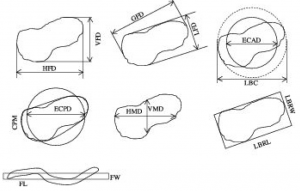Particles can exist in a myriad of different shapes. This can make conducting particle size analysis (PSA) through laser diffraction difficult as it assumes that particles are spherical. Some examples of minerals whose particles are extremely nonspherical include Wollastonite (rod-shaped) and Garnet crystals (cubic).

One important value to keep in mind when conducting PSA is called the aspect ratio, which is the ratio of the length to the width of a particle. Empirical analysis has shown that the smaller the aspect ratio of a particle, the more its true data will agree with those found using models that assume perfect spheres. The aspect ratio can be determined through scanning electron microscopy (SEM) of individual particles, and can be calculated in different ways. This is especially true of particles that have unclear lengths and widths, like the ones in the figure below. Some examples of calculations for the aspect ratio:

• The greatest Feret’s diameter (GFD) to the least Feret’s diameter (LFD)
• The lest bounding rectangle length (LBRL) to the least bounding rectangle width (LBRW)
• the fiber length (FL) to the fiber width (FW)

Examples of these and other methods of particle aspect ratio calculation can be seen hereIt is vital to keep particle shape in mind because the large majority of laser-based particle size analysis instruments rely heavily on methods that make the assumption of spherical particles. These methods primarily include the Mie and Frauenhofer methods. In order to account for biases within these methods, it is important to note the possible areas of a distribution that could be impacted. Some common discrepancies include an oversized distribution, meaning a distribution with an overly large width (showing larger and smaller particles than actually exists). This happens most commonly in samples with large aspect ratios.

In order to determine the impact of these biases, distributions can be compared to known standards. In order to obtain more accurate results, other characterization methods may be employed as well.

One common method (which is not available in the MCL), is the electrical sensing zone method. This uses an electrolytic solution in which the particles are suspended and which runs through an aperture. As the particles pass through the aperture, electric pulses are recorded and the concentration and volumes of the particles are measured. This method outperforms laser diffraction particle analysis when determining particle sizes of samples with large aspect ratios.